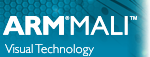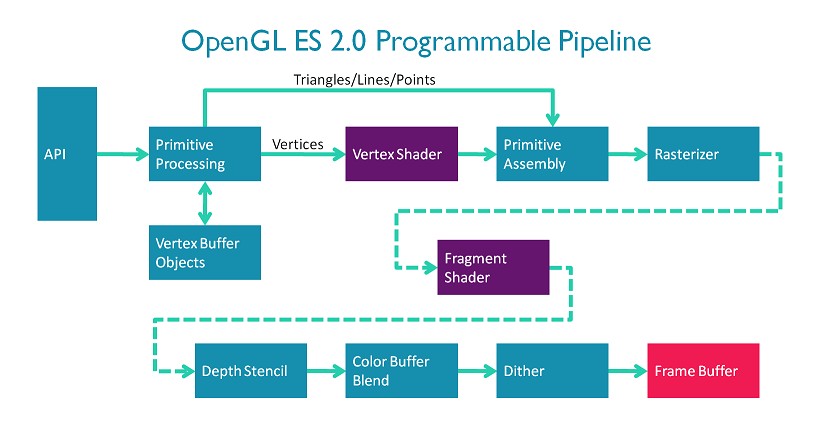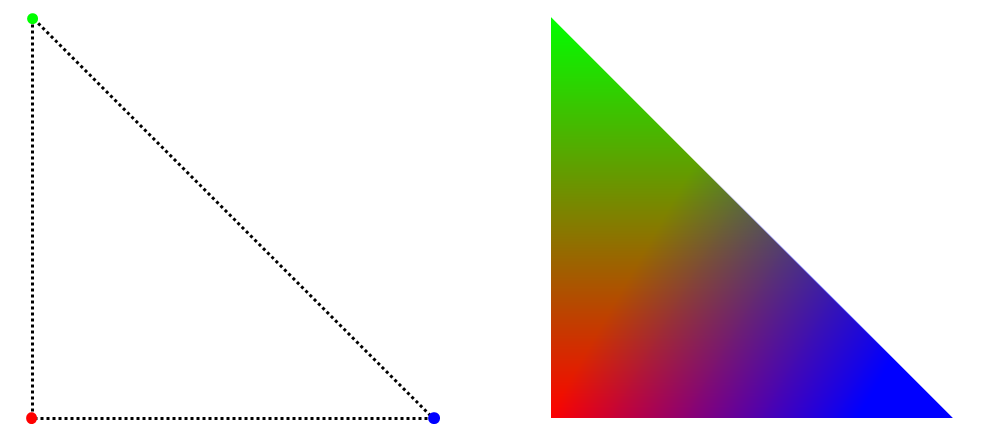OpenGL ES SDK for Android ARM Developer Center

A quick introduction to the programmable graphics pipeline introduced in OpenGL ES 2.0.

Introduction

This series of tutorials is going to focus on programmable graphics APIs. What does this mean? OpenGL ES 2.0 was the first OpenGL ES version to support a programmable graphics pipeline. Prior to this, we had OpenGL ES 1.x. OpenGL ES 1.x is a fixed function mobile graphics API. A fixed function graphics API allows you to specify various bits of data (positions, colours, etc.) for the GPU to work on, and a strict set of commands for using that data.

For example, you can tell the GPU that you have a polygon in your scene, specify the reflectance of the material for that polygon, and add a light to the scene. The GPU will then go a away and calculate what colour the polygon will appear in your scene.

This is great for simple scenes and allows you to very quickly achieve some very nice looking graphical effects.

However, what happens when you want to use a different lighting equation that the one built in to the API? What if you come up with a great new graphics technique but the API doesn't support it? (e.g. frame buffer objects, cube maps, etc.). You're very dependent on the contents of the API; there are a fixed set of functions and those functions have a limited number of options. This is never going to cover all the things you might want to do as a developer. And although the API can be extended to support more options and more techniques, you're then dependent on GPU vendors implementing those extensions.

That's where fixed function graphics pipelines fall short. Instead of creating hundreds of extensions and functions to support all requested use cases, programmable pipelines were created. A programmable pipeline allows the developer to provide custom code that is run on the GPU at various stages of the pipeline. Each piece of code is called a shader, and since they can contain arbitrary code it means the developer is free to do (almost) whatever they like.

Of course, all this freedom comes with a price. Since there are no default shaders in OpenGL ES 2.0 you must always write your own. This means that features like lighting, texturing, and others that are "free" (in terms of developer effort) in OpenGL ES 1.1 must now be programmed explicitly by the developer.

It's worth mentioning that OpenGL ES 1.x and OpenGL 2.0 onwards cannot be mixed and OpenGL ES 2.0 is not backwards compatible. In other words, it's all or nothing when choosing fixed function vs. programmable.

Portability

Since shaders are C-like programs which run on a GPU, you may be thinking, "how do I compile these shaders?". Because each GPU vendor (and sometimes each GPU) has a different instruction set we need a way to compile the shaders for every different device.

Thankfully this is possible in OpenGL ES using "online" compilation. You provide the source code for you shaders to the OpenGL ES API at runtime and the OpenGL ES driver uses a compiler on the device itself to compile your code for instruction set of the device.

// Create a shader object (we'll see the types of shader you can create later).
// Add the source code to the shader object.
// Compile the shader source code.

Shaders work together in programs. This is similar to what happens when compiling normal C code: you compile your source to object files (.o) and then link them together into an executable. Here, you compile you shaders, add them to a program and then link them together.

// Create a program object.
glCreateProgram();
// Attach shader objects to that program.
// Link the shaders objects inside a program.

This leaves you with a program object which you can use in your application.

// Tell OpenGL ES which program you want to use.
glUseProgram( GLuint program);

The downside here is of course that anybody who wants to will be able to see you shader code which will contain your top secret super-fancy graphics techniques. In the interest of keeping your shader source code propriety, most GPU vendors also provide an "offline" compiler which can generate binaries for their particular platform. These binaries can then be shipped with you application and loaded using the OpenGL ES API at runtime. You will have to create and ship binaries for each platform you wish to run on and pick the correct one.

You can load binaries by swapping glShaderSource above for glShaderBinary.

The OpenGL ES Programmable Pipeline

A diagram of the OpenGL ES 2.0 pipeline can be found below.The OpenGL ES Programmable Pipeline

The vertex and fragment stages are the programmable stages in the OpenGL ES 2.0.

The code you write in the vertex shader will be run once per vertex. So if you tell OpenGL ES to draw ten unconnected triangles, your vertex shader will run 30 times.

The only required job of the vertex shader is to emit the final position of the vertex.

In a very trivial (and useless) example, your vertex shader could simply set the position of each vertex to (0, 0, 0). This doesn't require any data to be passed in to the shader. The #version 100 here tells OpenGL ES that we are using version 1 of the OpenGL ES Shader Language.

#version 100
// Trivial vertex shader.
void main()
{
gl_Position = vec4(0.0, 0.0, 0.0, 1.0);
}

More normally, you would pass in the coordinates of the shapes you want to draw and use these as your positions. For this you need to pass in some data for each vertex you want to draw. This type of per vertex data is handled in OpenGL ES using vertex attributes.

If you want to draw a single triangle, you need three vertices. Let's say (1.0, 0.0, 0.0), (0.0, 1.0, 0.0), and (-1.0, 0.0, 0.0).

To get this data in, we can pass the array [1.0, 0.0, 0.0, 0.0, 1.0, 0.0, -1.0, 0.0, 0.0] to OpenGL ES and associate it with a 3-component attribute (e.g. a vec3). When you tell OpenGL ES you want to draw a single triangle, it will split up the array and pass each 3-component position to a different vertex shader.

We can then use this data to set the position of the vertex in the vertex shader.

// Host code to set data up to be used in the shader.
// Create you C array of vertex positions.
GLfloat [] positions = {1.0, 0.0, 0.0, 0.0, 1.0, 0.0, -1.0, 0.0, 0.0};
// To associate this data with a particular input to the shader, we must get that inputs "location" in the shader. Note, this can only be done after the glProgram has been linked.
GLint position_location = glGetAttribLocation("position");
// Finally, tell OpenGL ES to use the 'positions' array as the input data.
glVertexAttribPointer(position_location, ..., positions);
#version 100
// Vertex shader which takes position as a per-vertex input.
// Inputs
attribute vec4 position;
void main()
{
gl_Position = position;
}

Great, we can now pass data in to a vertex shader and set a sensible position. The nice thing is, that we can use these same techniques to pass in any data we want to be different per vertex. So if we want to have a colour associated with each vertex we can do that too.

// Same code as before to set data up to be used in the shader.
GLfloat [] colours = {1.0, 0.0, 0.0, 0.0, 1.0, 0.0, 1.0, 0.0, 0.0};
GLint colour_location = glGetAttribLocation("colour");
glVertexAttribPointer(colour_location, ..., colours);
#version 100
// Vertex shader that takes in per-vertex position and colour inputs.
// Inputs
attribute vec4 position;
attribute vec4 colour;
void main()
{
gl_Position = position;
}

Now you're thinking what good is a colour in the vertex shader if the only thing I can output is a vertex position. Well, it turns out you can also create arbitrary outputs from a vertex shader which will be interpolated across the shape you are drawing. These are called varyings. The varyings you output must exactly match the varyings you specify as inputs to the fragment shader.

#version 100
// Vertex shader that takes in per-vertex colour data and outputs it as interpolated per-fragment data.
// Inputs
attribute vec4 position;
attribute vec4 colour;
// Outputs
varying vec4 interpolated_colour;
void main()
{
interpolated_colour = colour;
gl_Position = position;
}

The Rasterizer

There are two stages in our pipeline diagram in between the vertex and fragment stages: primitive assembly and rasterization. Since all modern display technologies are raster based (they can display only coloured points), we need some way to turn our concept of points and triangles into points on the screen. The rasterization step does this part for us, working out which primitives cover which points and then generating fragment jobs for those points. As it generates the fragment job it calculates the interpolated values for our varyings using something called barycentric coordinates. We won't go into the details here but in the example below, on the left side we have a triangle with red, green, and blue specified as the colour attributes for the three vertices. On the right the colours have been interpolated across the triangle.Interpolating per-vertex colours across a triangle.

That's an easy to visualise example using colours, but this technique works for all kinds of things.

This leads us nicely onto the fragment shader. The fragment shader is run (you guessed it!) once per fragment issued by the rasterizer.

The only output a fragment shader needs to emit is the colour of the fragment. So to give another trivial example we could just set the colour of every fragment to black: (0.0, 0.0, 0.0, 1.0).

#version 100
// Trivial fragment shader.
void main()
{
gl_FragColor = vec4(0.0, 0.0, 0.0, 1.0);
}

But that's no fun.

So since we've passed some interpolated colours from the vertex shader, we can use those here.

#version 100
// Fragment shader which takes interpolated colours and uses them to set the final fragment colour.
// Floating point values in fragment shaders must have a precision set.
// This can be done globally (as done here) or per variable.
precision mediump float;
// Inputs
varying vec4 interpolated_colour;
void main()
{
gl_FragColor = interpolated_colour;
}

Uniforms

So to recap so far we've covered:

• How to get per-vertex data into the fragment shader
• How to get interpolated per fragment data into the fragment shader

What about constant data? What if we have some data which is common to all vertices, or to all fragments or to everything?

This could be anything from a time value to something describing the level of light in the scene.

A very common use for this would be having a transformation matrix which positions you vertices in a scene. You need the same matrix for each vertex so that they all get transformed together.

For this constant type of data we have shader uniforms. For the most part declaring, setting and using uniforms is the same as attributes except that you only set one set of values.

For example:

#version 100
// Vertex shader which takes a constant input value (a uniform) and uses it to alter all vertex x positions.
// Per-vertex inputs
attribute vec4 position;
attribute vec4 colour;
// Constant inputs
uniform int x_offset;
// Outputs
varying vec4 interpolated_colour;
void main()
{
interpolated_colour = colour;
gl_Position = position + vec4(x_offset, 0.0, 0.0, 0.0);
}

More differences between attributes and uniforms are listed in Appendix: Attributes vs. Uniforms.

Textures

There's one last type of data that we could need, per-fragment data that is not interpolated from per-vertex data. For example, what if we want to display an image on a triangle. We can't use uniforms since we don't typically have enough to store all the pixels in an image(not to mention that setting and accessing the data would be awful). We also can't use attributes and varyings since those are per-vertex and then interpolated which doesn't make sense for an image. Luckily for us OpenGL ES has this covered too. For this purpose we use textures.

Textures allow us to specify arbitrary arrays of data which can be accessed in the fragment shader.

How does each fragment shader instance know where to look in the texture? Good question, but we already have all the knowledge we need to solve this problem. If we specify where in the image each vertex maps to (using an attribute), the rasterizer will interpolate the coordinates nicely for us to give us a per-fragment varying. This coordinate can then be used to look up the correct point in the image. This is done using a texture sampler.

#version 100
// Vertex shader which takes a per-vertex texture coordinate and interpolates it for the fragment shader.
attribute vec4 position;
attribute vec2 texture_coordinates;
varying vec2 interpolated_texture_coordinates;
void main()
{
interpolated_texture_coordinates = texture_coordinates;
gl_Position = position;
}
// Host side code to set up texture input.
Glint sampler_location = glGetUniformLocation(glProgram, "texture_sampler");
// ...
// Create a Texture (this can be quite involved so we won't go into detail here but it's basically uploading an array of data similar to vertex attributes.)
// ...
// Set the sampler to point to the texture you've just created.
glUniform1i(sampler_location, texture_unit);
#version 100
// Fragment shader which looks inside a texture using interpolated texture coordinates.
precision mediump float;
varying vec2 interpolated_texture_coordinates;
uniform sampler2D texture_sampler;
void main()
{
gl_FragColor = texture2D(texture_sampler, interpolated_texture_coordinates);
}

And now the Fun Begins!

We've focused on very trivial examples here just to expose the data flows in OpenGL ES. It might not have sold you on why you would want this, or why should you care. Well, this is really only the ground layer. OpenGL ES shaders also allow you do general purpose maths (including lots of hardware accelerated built-in functions). This fact, combined with the data flows we've already covered gives you the ability to harness the power of a massively parallel computing unit: the GPU.

Because of the flexibility afforded to you by the OpenGL ES 2.0 API you're free to implement whatever graphical effects you can imagine. Almost all high-end 3D graphics applications (e.g. games) will be using the basic methods described above; using shaders to achieve their effects.

Summary

So in summary we have seen the OpenGL ES programmable pipeline and introduced the two shader types available in OpenGL ES 2.0: vertex and fragment shaders.

Vertex shaders have the following interface:

• Vertex attributes (per-vertex data) and uniforms (constant data) comes in.
• Vertex positions and varyings go out.

Fragment shaders have the following interface:

• Varyings(interpolated per-fragment data), uniforms (constant data), and textures comes in.
• Fragment colours go out.

This was a very quick introduction to shaders and the programmable pipeline in OpenGL ES. Don't worry if you didn't quite catch all the details (we haven't really begun to scratch the surface here). The following tutorials go into far more detail and use practical examples (with working source code) to further explain these concepts.

Next Steps

Now move on to Graphics Setup.

Appendix: Attributes vs. Uniforms

Some further differences between uniforms and attributes for reference:

Accessibility:

• Attributes are values which are only available in the vertex shader (although you can pass them on the the fragment shader as we we see later).
• Uniforms can be accessed in either the fragment or vertex shader.

Type of data:

• Attributes are values which vary per vertex.
• Uniforms are constant values that are the same for all instances of the fragment and vertex shaders.

Object model:

• Attributes are attached to locations.
• Uniforms are attached to program objects.

Availability:

• There are (generally) less attributes available than uniforms (although both are implementation defined). You can find out the exact number supported on your platform by doing glGet(...) with the appropriate parameter (found in brackets below).
• An implementation must support at least 8 vertex attributes (GL_MAX_VERTEX_ATTRIBS).
• An implementation must support at least 128 vertex uniforms (GL_MAX_VERTEX_UNIFORM_VECTORS).
• An implementation must support at least 16 fragment uniforms (GL_MAX_FRAGMENT_UNIFORM_VECTORS).
• OpenGL ES has various other implementation defined properties which can be queried using glGet(...). Some relavent ones for this introduction are:
• An implementation must support at least 8 varyings (GL_MAX_VARYING_VECTORS).
• An implementation must support textures of at least size 64 varyings (GL_MAX_TEXTURE_SIZE).